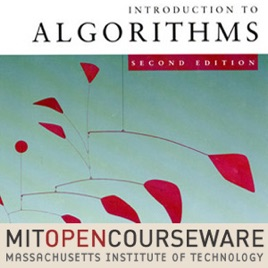Выпусков: 23

This course teaches techniques for the design and analysis of efficient algorithms, emphasizing methods useful in practice. Topics covered include: sorting; search trees, heaps, and hashing; divide-and-conquer; dynamic programming; amortized analysis; graph algorithms; shortest paths; network flow; computational geometry; number-theoretic algorithms; polynomial and matrix calculations; caching; and parallel computing.# Introduction to Algorithms (2005) MIT

• Технология

This course teaches techniques for the design and analysis of efficient algorithms, emphasizing methods useful in practice. Topics covered include: sorting; search trees, heaps, and hashing; divide-and-conquer; dynamic programming; amortized analysis; graph algorithms; shortest paths; network flow; computational geometry; number-theoretic algorithms; polynomial and matrix calculations; caching; and parallel computing.

4.7 из 5
Оценок: 18

Оценок: 18